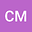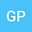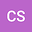Neimark-Sacker, flip and transcritical bifurcation in a symmetric system of difference equations with exponential terms
•••Chrysoula Mylona
Democritus University of Thrace

Corresponding Author:cmylona@ee.duth.gr

Author ProfileChristos Schinas
Democritus Univ. of Thrace
Author Profile## Abstract

In this paper, we study the conditions under which the following symmetric system of difference equations with exponential terms: $x_{n+1} =a_1\frac{y_n}{b_1+y_n} +c_1\frac{x_ne^{k_1-d_1x_n}}{1+e^{k_1-d_1x_n}},$ $y_{n+1} =a_2\frac{x_n}{b_2+x_n} +c_2\frac{y_ne^{k_2-d_2y_n}}{1+e^{k_2-d_2y_n}}$ where $a_i$, $b_i$, $c_i$, $d_i$, $k_i$, for $i=1,2$, are real constants and the initial values $x_0$, $y_0$ are real numbers, undergoes Neimark-Sacker, flip and transcritical bifurcation. The analysis is conducted applying center manifold theory and the normal form bifurcation analysis.
09 Feb 2021Submitted to Mathematical Methods in the Applied Sciences
10 Feb 2021Submission Checks Completed
10 Feb 2021Assigned to Editor
15 Feb 2021Reviewer(s) Assigned
28 Feb 2021Review(s) Completed, Editorial Evaluation Pending
01 Mar 2021Editorial Decision: Revise Minor